# What is a factor?

In this guide, learn how factors ‘factor’ into all areas of maths!Author
Taylor HartleyExpert Reviewer

Published: November 3, 2023# What is a factor?

In this guide, learn how factors ‘factor’ into all areas of maths!Author
Taylor HartleyExpert reviewer

Published: November 3, 2023# What is a factor?

In this guide, learn how factors ‘factor’ into all areas of maths!Author
Taylor HartleyExpert reviewer

Published: November 3, 2023Key takeaways

• Factors divide a number evenly: when dividing a number (dividend) by another number (divisor), you can get whole numbers or numbers with reminders. The divisor that gets you a whole number is a factor!
• You’ll use factors a lot: whether you’re trying to simplify fractions or make division problems more manageable, understanding factors will help you in many areas of maths.
• Factors have to be whole numbers: fractions aren’t allowed in the world of factors.

Factors are a nice first step in seeing the many ways different maths rules and concepts overlap. When you find the factors of numbers, you’ll begin to notice patterns. These patterns, and the process of finding fractions itself, reveal just how interconnected everything you are learning about maths is!

## What is a factor?

In short, factors are whole numbers that you can divide another number by and get a whole quotient.

Remember, a whole quotient is an answer that is a complete number with no remainder. Every number has its own set of factors, and you find factors the same way for all numbers.

When beginning to think about factors, it helps to look at numbers as groups of other numbers. For example, you can split 12 into one group of 12, two groups of 6, or three groups of 4.

Sometimes, thinking about objects can make this process easier to visualise. Let’s imagine 12 ducks hanging out on a pond. Those ducks can float around in groups of 1, 2, 3, 4, 6, and 12, and not a single quacker would be left out!

That’s basically what factoring is: thinking about the different ways you can divide a starting number without getting a remainder.

Based on the example above, the numbers 1, 2, 3, 4, 6, and 12 are all factors of 12. This is because when you divide 12 by any of these numbers, the answer is whole. 12 ÷ 2 = 6, 12 ÷ 3 = 4, and so on.

## A few notes on factors

For all numbers, 1 and the number itself are technically factors.

For example, with the number 54, both 1 and 54 are factors. Because this rule is true for every number, you may see problems where 1 and the number itself are not included when listing out factors.

We’ll include them as a reminder that they are factors, but remember, you may not see them listed as factors elsewhere.

If you’re looking for a way to remember what a factor is, think about the word ‘factory’. They sound pretty similar, right? Well, that’s because they both come from the Latin word for ‘make’! Factories make things, and factors make up other numbers. If you make that connection in your head, you’ll have a much easier time remembering the ins and outs of factors.

### Prime and composite numbers

There’s one more thing to cover before we dive into exactly how you find the factors of a number. When finding these factors, you’re going to be finding prime and composite numbers.

What does this mean? Well, prime numbers are numbers that are only divisible by 1 and themselves. For instance, the number 3 is only divisible by 1 and 3, so it is a prime number.

The number 1, however, is the sole exception to this rule. This is because 1 is technically not a prime number. 1 is divisible by 1, but that is all. For a number to be prime, it needs to be divisible by 1 and at least one other number. That means 1 does not count.

Composite numbers are numbers with more than two factors. Basically, if a number is not prime, it is probably composite.

The main exceptions to these rules are the numbers 1 and 0.

## Explore factors with DoodleMaths

Want to learn more about factors? DoodleMaths is an app that’s filled with games and questions exploring multiplication, shapes and more!

Designed to be used ‘little and often’, it creates each child a unique work programme tailored to their needs, boosting their confidence and ability in maths.

Best of all, it sets work at just the right level of challenge, letting every child work independently. Try it for free today!## How to factorise

Now that you know the basics, let’s get to factoring!

The most straightforward way to find factors is to start with the dividend, or the number that will be divided. Then, go through each counting number and see which ones the dividend is evenly divisible by. Continue this process all the way up to the number itself. Let’s see this in action.

Okay, what are the factors of 16? Well, we know that all numbers are divisible by 1 and themselves, so 1 and 16 are factors of 16. Now we just need to check all of those between 1 and 16.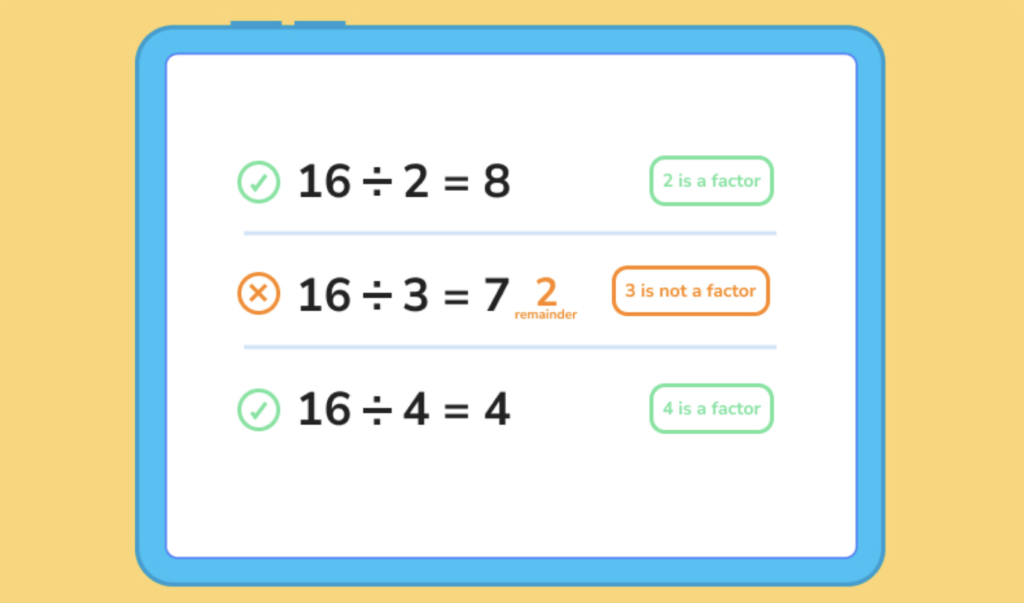Simply follow this process until you get to 12!

This leaves you with the factors 1, 2, 4, 6, 8, and 16. Remember the section about prime and composite numbers? 2 is prime because it is divisible by just 1 and 2 (itself). The rest of the factors are composite.

### That took a long time...

Factoring this way takes a little bit of time. To speed things up, let’s learn about those patterns mentioned at the beginning of the lesson. There’s sort of a secret code in maths that allows you to anticipate patterns, and this is true to factoring.

For example, if the number is even, you know it has to be divisible by 2, which means 2 is a factor.

The answer you get when you divide by 2 is also a factor. Just look at the factors of 16 above. Since 2 x 8 = 16, both 2 and 8 are factors.

Aside from the number itself, there will never be a factor greater than the number divided by 2. For instance, 16 is largest possible factor (besides itself), is 8.  Therefore, you can stop checking for factors once you get to 8, saving yourself a whole lot of time.

Additionally, if the dividend is not even, you know none of the factors will be even either. So, you can skip trying to divide any even number into an odd number, cancelling out half of your possible factors!

Here’s another trick: if the digits in the dividend add up to a number divisible by 3, the entire number is divisible by 3!

For example, the number 132. 1 + 3 + 2 = 6. 6 is divisible by 3, so 132 is also divisible by 3. Additionally, just like with 2, if you divide by 3, the answer you get is a factor. This is true for any factor you find!

Here’s one more trick: any number that ends in 0 or 5 will be divisible by 5, and any number that ends in 0 is divisible by 10.

With these tricks and patterns in mind, you can quickly find the factors of any number.

### Factoring large numbers

When factoring large numbers, it’ll take a super long time to test every individual number. Instead, factor out the factors you have already found until you’re left with nothing but prime numbers.

For example, if you wanted to factorise 81, you could easily get 1, 3, 27, and 81 using the tricks we previously covered. After that, you factor the factors! 27 can be factorised into 3 and 9, and since 9 is a factor of 27, it is also a factor of 81.

Factoring all the factors until you are left with nothing but prime numbers is called prime factorisation. Prime factorisation is key to helping you find the factors of larger numbers.

## How to find factors: examples

### 1. What are the factors of 18?

• 1 and 18 are obviously factors, so we can go ahead and write those down.
• 18 is an even number, so 2 is a factor. 18 ÷ 2 = 9, so 9 is a factor.
Remembering our tricks, we also know that no factors can be greater than 9.
• 1 + 8 = 9, which is divisible by 3, so 3 is a factor. 18 ÷ 3 = 6, so 6 is a factor.
• 18 doesn’t end with 0 or 5, so 5 and 10 aren’t factors.
• That means we have 1, 2, 3, 6, 9, and 18 as factors. Now we just have 4, 7, and 8 to test.
• 18 ÷ 4 = 16 remainder 2, so no to that one.
• 18 ÷ 7 = 14 remainder 4. That doesn’t work either.
• 18 ÷ 8 = 16 remainder 2. No good!
• That means our factors of 18 are 1, 2, 3, 6, 9, and 18!

### 2. What are the factors of 25?

• 1 and 25, of course.
• 25 is odd, so 2 (and all other even numbers) are out.
• 2 + 5 = 7, which is not divisible by 3.
• 25 ends in 5, so 5 is a factor. Let’s divide by 5 to get one more factor. 25 ÷ 5 = 5.
• We have 1, 5, and 25!

### 3. What are the factors of 29?

• 1 and 29.
• 29 is odd, so no to 2 and all other even numbers.
• 2 + 9 = 11, which is not divisible by 3.
• 29 does not end in 0 or 5, either.
• We still need to check other odd numbers like 7, 9, 11, 13, and 15. You can think through your multiplication tables to see that none of them go evenly into 29.
• That leaves us with 1 and 29, which means 29 is prime.

## Factor practice questions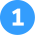Find the factors of 23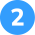Find the factors of 72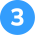Find the factors of 38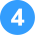Which number is prime, 67 or 90?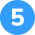Find the factors of 1001 and 23

How did we get here?

1. 1 and 23 are obviously factors, so we can go ahead and write those down.
2. 23 is not an even number, so 2 is not a factor. This also means no other even number will be a factor.
3. 2 + 3 = 5, which is not divisible by 3, so 3 is not a factor.
4. 23 does not end with 0 or 5, so 5 and 10 are not factors.
5. We still need to check other odd numbers like 7, 9, 11, and 13. You can think through your multiplication tables to find that none of them divide evenly into 23.
6. That means our factors of 23 are just 1 and 23. 23 is prime!1, 2, 3, 4, 6, 8, 9, 12, 18, 24, 36, and 72

How did we get here?

1. 1 and 72 are obviously factors.
2. 72 is an even number, so 2 is a factor. 72 ÷ 2 = 36, so 36 is a factor. We also know that no factors can be greater than 36.
3. 7 + 2 = 9, which is divisible by 3, so 3 is a factor. 72 ÷ 3 = 24, so 24 is a factor.
4. 72 does not end with 0 or 5, so 5 and 10 are not factors.
5. That means we have 1, 2, 3, 24, 36 and 72 as factors. Now we need to use prime factorization to find  the rest.
6. 24 has factors of 1, 2, 3, 4, 6, 8, 12, and 24, so these are all factors of 72. 4, 6, 8, and 12 are all new factors here, so we can add them to our list.
7. Factoring out 36 gets us 1, 2, 3, 4, 6, 9, 12, 18, and 36. 18 is the only new factor here, so we can add it to our list.
8. That means our factors of 72 are 1, 2, 3, 4, 6, 8, 9, 12, 18, 24, 36, and 72.1, 2, 19, and 38

How did we get here?

1. 1 and 38 are obviously factors.
2. 38 is an even number, so 2 is a factor. 38 ÷ 2 = 19, so 19 is a factor, and no factors can be greater than 19.
3. 3 + 8 = 11, which is not divisible by 3, so 3 is not a factor.
4. 38 does not end with 0 or 5, so 5 and 10 are not factors.
5. That means we have 1, 2, 19, and 38, and need to use prime factorization to get the rest.
6. 2 and 19 are already prime, so there are no new factors.
7. That means our factors are 1, 2, 19, and 38.67 is prime

How did we get here?

1. Factor out both numbers as normal.
2. 90 has more than two factors, so it is not prime.
3. 67 only has the factors of 1 and 67, so it is prime.1, 2, 4, 5, 10, 20, 25, 50, and 100

How did we get here?

1. 1 and 100 are obviously factors.
2. 100 is an even number, so 2 is a factor. 100 ÷ 2 = 50, so 50 is a factor, and no factors can be greater than 50.
3. 1 + 0 + 0 = 1, which is not divisible by 3, so 3 is not a factor.
4. 100 ends with 0, so 5 and 10 are factors. 100 ÷ 5 = 20, and 100 ÷ 10 = 10. So, 5, 10, and 20 are factors.
5. After factoring more we have 1, 2, 4, 5, 10, 20, 25, 50 and 100. Now we can use prime factorization to get the rest.
6. That process doesn’t get us any new factors, so we are all set!

## FAQs about factors for kids

In maths, a factor is a number you can use to divide another number without any remainder.

Go through all the counting numbers and use them to divide the number you’re trying to factorise.

Each counting number that divides the starting number (or the dividend) into a whole number is a factor.

Look for even numbers, numbers where the digits add up to a number divisible by 3, and numbers that end in 0 and 5. These can all be quickly divided to find many factors.

Prime numbers are numbers that can be factored only into 1 and the number itself. 1 doesn’t count as a prime number.## Related Posts

Title 1

Title 2

Title 3Lesson credits

Taylor Hartley

Taylor Hartley is an author and an English teacher based in Charlotte, North Carolina. When she's not writing, you can find her on the rowing machine or lost in a good novel.

Jill Padfield has 7 years of experience teaching mathematics. She is currently working as a Business Analyst, working to improve services for Veterans while earning a masters degree in business administration.

Taylor Hartley

Taylor Hartley is an author and an English teacher based in Charlotte, North Carolina. When she's not writing, you can find her on the rowing machine or lost in a good novel.

Jill Padfield has 7 years of experience teaching mathematics. She is currently working as a Business Analyst, working to improve services for Veterans while earning a masters degree in business administration.

## Related posts

How to multiply fractions

Take your learning further by exploring how to multiply fractions

What are odd and even numbers?

We explore examples of odd and even numbers from 1 to 100

How to improve at mental maths​

Learn some handy mental maths strategies that’ll make addition a breeze!

# Are you a parent, teacher or student?

Are you a parent or teacher?

## Hi there!

Book a chat with our team